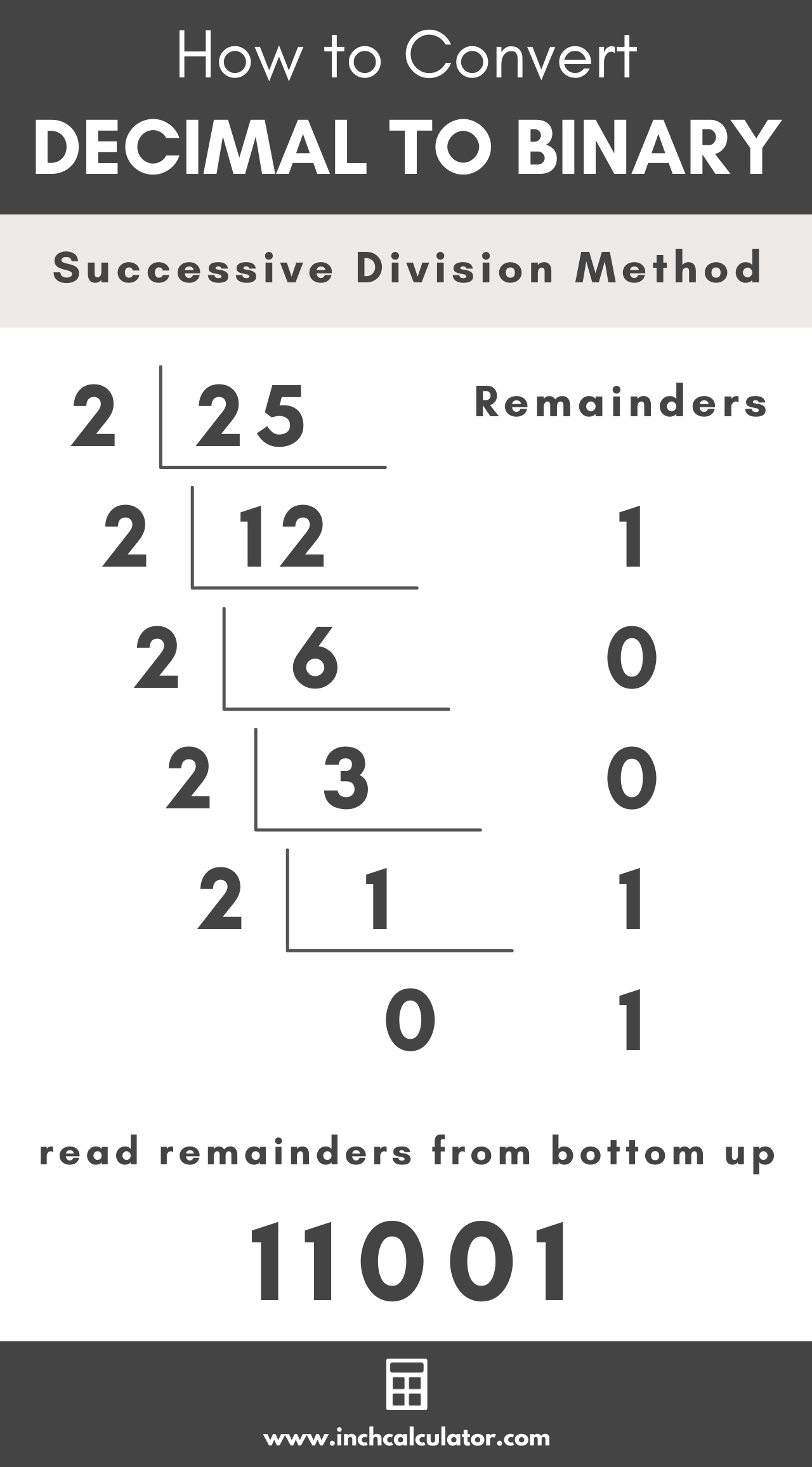# Decimal to Binary Converter

Enter a decimal number below to convert it to a binary.

## Binary Number:

### Steps to Convert to Binary

Learn how we calculated this below

## How to Convert Decimal to Binary

The decimal number system is the number system most of us use every day. The decimal system, also referred to as denary, is a base 10 number system, which means it is composed of 10 digits, 0, 1, 2, 3, 4, 5, 6, 7, 8 & 9.

Binary numbers, also referred to as base 2 numbers, are the foundation of computing systems because they consist of only two digits, 0 and 1. This allows them to easily be used with electrical and mechanical switches. Binary numbers can also be used with modern transistors that make up modern computer processors.

You might be wondering how to convert from a decimal number to a binary number when these number systems do not use the same digits. To answer this, we need to dig in a little bit on how a binary number works.

A binary number is read from right to left, with the least significant bit being the digit on the far right end of the number and the most significant bit being the digit on the far left end.

### Digit Values in a Binary Number

Each digit can be only a 1 or a 0, and digits that are a 1 are equivalent to 2n-1 where n is equal to the distance from the right side of the number.

This table shows the decimal values of the first nine digits in a binary number. Of course, a binary number can be much larger than nine digits, and the decimal value grows exponentially with each digit.

Table showing decimal values of the first nine digits in a binary number.
MSB Binary Digit LSB
28 27 26 25 24 23 22 21 20
256 128 64 32 16 8 4 2 1

### Steps to Convert

Now that you understand the representation of a base 2 number, you can convert from decimal to binary using successive division by 2. Whoa! I bet you’re probably wondering what that means!.

To use the successive division by two method, divide the decimal number by 2 using long division. There should be a remainder of 0 or 1; write that to the side of the division problem.

Take the result of the first division problem and divide that by 2 again. Like before, there should be a remainder of 0 or 1, which you should write to the side of the problem.

Continue this process until the result is 0.

The remainders that you wrote to the side of the division problems are the resulting binary number. The number should be read from the bottom to the top since the least significant digit will be at the top, and the most significant digit will be at the bottom.For example, let’s convert 25 from base 10 to base 2.

25 ÷ 2 = 12 R 1 (LSB)
12 ÷ 2 = 6 R 0
6 ÷ 2 = 3 R 0
3 ÷ 2 = 1 R 1
1 ÷ 2 = 0 R 1 (MSB)

Reading the remainders from the bottom up is 11001, so 2510 is equivalent to 110012.

### Decimal to Binary Conversion Video Tutorial

Want to convert back to decimal to confirm your answer? Try our binary to decimal converter.

## How to Convert a Fraction to Binary

To convert fractional decimal value to binary, multiply the fractional portion of the decimal by 2. If the result is greater than 1, then subtract 1 from the result and note that the binary bit will be a 1; otherwise, the binary bit will be a 0.

Continue multiplying by 2 until the resulting decimal value is equal to zero. The fractional result in binary is the remainder 0 and 1 values in order.

For example, let’s convert 0.25 from decimal to binary.

0.25 × 2 = 0.5 + 0
0.5 × 2 = 0 + 1

So, the fractional binary value is equal to 0.01

## Decimal to Binary Conversion Table

The table below shows decimal numbers and the equivalent binary number values.

Decimal numbers converted to the equivalent binary values
Decimal Number Binary Number
0 0
1 1
2 10
3 11
4 100
5 101
6 110
7 111
8 1000
9 1001
10 1010
11 1011
12 1100
13 1101
14 1110
15 1111
16 10000
17 10001
18 10010
19 10011
20 10100
21 10101
22 10110
23 10111
24 11000
25 11001
26 11010
27 11011
28 11100
29 11101
30 11110
31 11111
32 100000
64 1000000
128 10000000
256 100000000
512 1000000000
1024 10000000000
2048 100000000000